# Creating/Modifying Angle DimensionsThis task will show you how to create an angle dimension and perform the following kinds of modifications: new angle sector or turn an angle sector into a supplementary sector.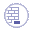Create two lines.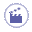1. Click Dimensions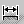in the Dimensioning toolbar.

2. Select both lines to be dimensioned, one after the other.
The angle dimension appears in the sector associated to both selected lines.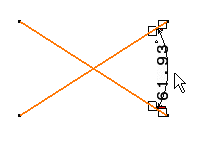3. Drag the angle dimension line to the desired quadrant (or sector).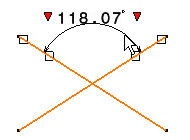You can move the dimension to a new sector by using the contextual menu:
• Right-click the angle dimension and from the contextual menu, Angle Sector, either select a given angle sector or the Complementary Angle sector.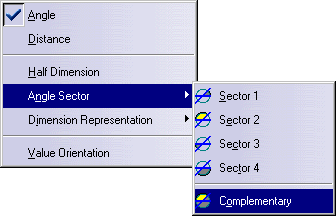• You can also CTRL-click the dimension line.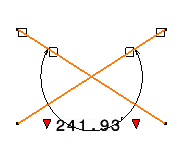1. Click anywhere to create the angle dimension.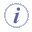You can edit the angle sector of an existing angle dimension, by right-clicking the angle dimension and selecting the Dimension_name object > Angle Sector command from the contextual menu.
2. Create a rectangle.

3. Select Angle Dimensions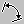in the Dimensioning toolbar.

4. Select two lines in the rectangle to create an angle dimension.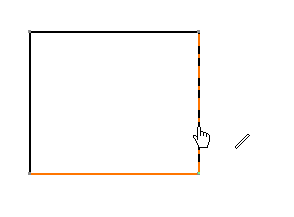5. Click to create the angle dimension, then right-click the dimension and select Half Dimension.

6. Click in a free space to create the dimension.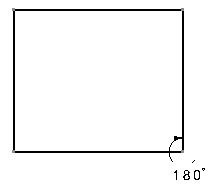Note that the half angle dimension's orientation depends on the order of selection of the lines to be dimensioned.

If you had you selected the vertical line first, then the horizontal one, the orientation of the dimension would had been the following: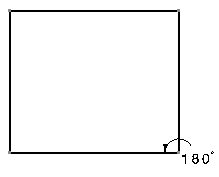If you need to modify the dimension, use Re-route dimension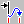.